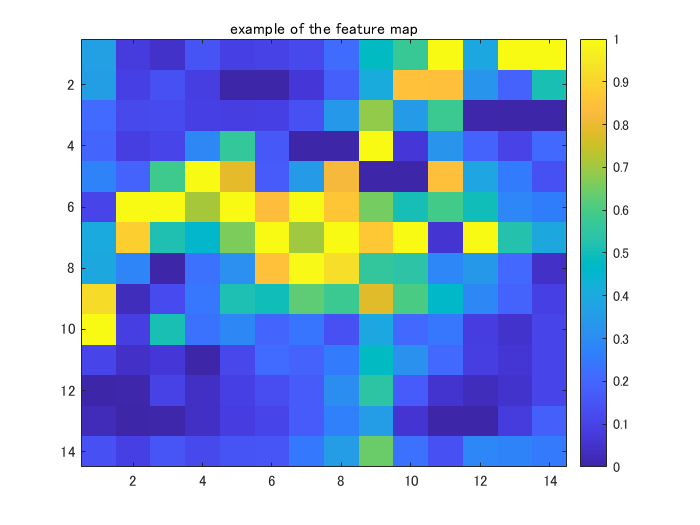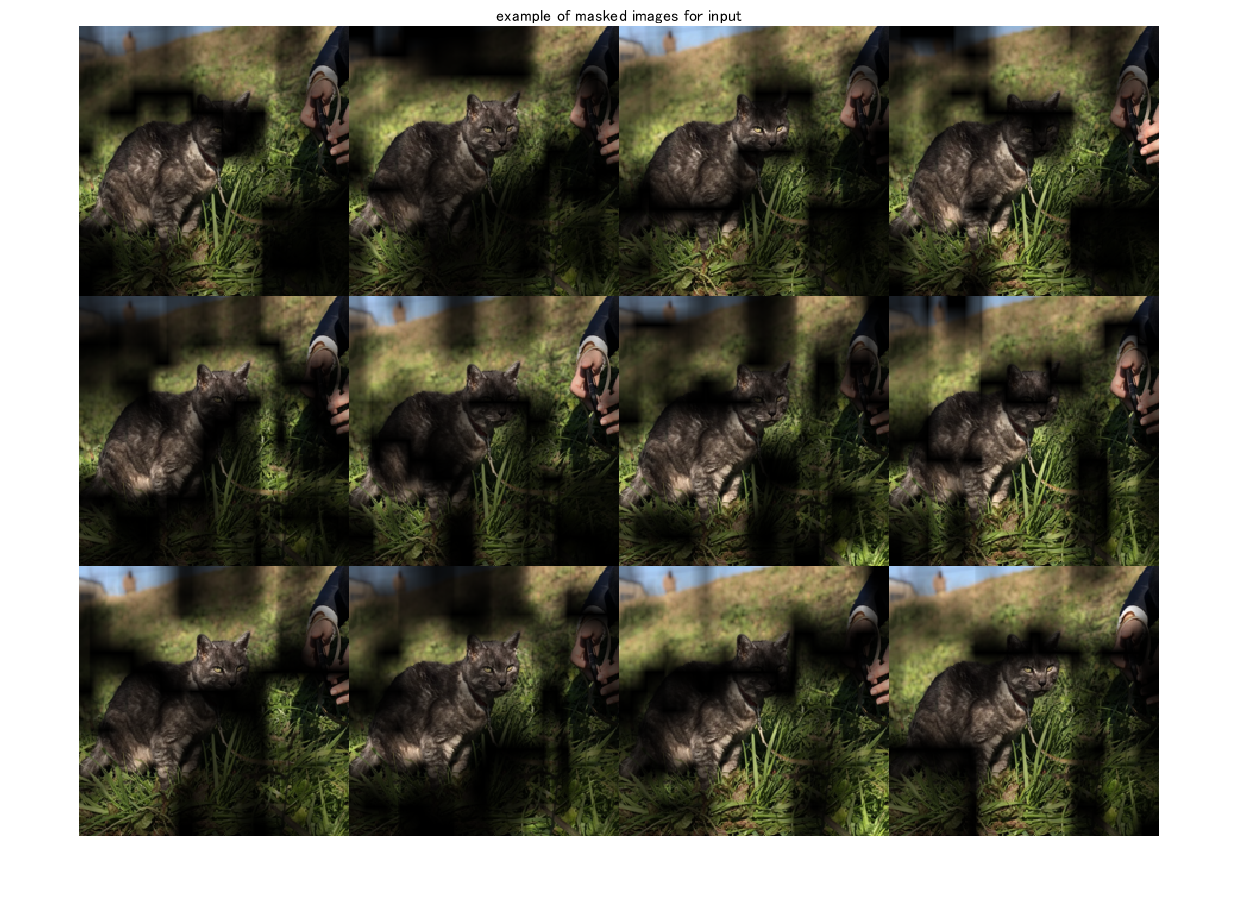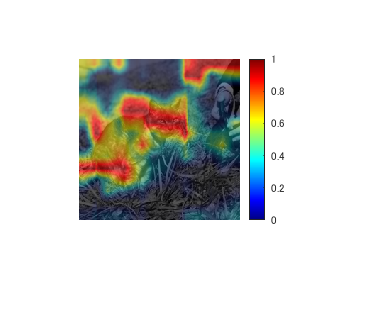## Explainable AI with Score-CAM

버전 1.1.0 (13.3 MB) 작성자:
This repo shows a MATLAB implementation of score-CAM (Wang et al., CVPR workshop, 2020). This method is the first gradient-free CAM-based vi

다운로드 수: 152

업데이트 날짜: 2021/12/16

GitHub에서 호스트# Explainable AI: interpreting the classification using score-CAM

[English]

This example shows how to use score-CAM (class activation mapping)  to investigate and explain the predictions of a deep convolutional neural network for image classification. The algorithm like CAM  and grad-CAM  are popular for explainable AI with image classification, but Score-CAM proposed a "gradient-free" method for the explanation. The original implementation can be found here.

[Japanese]

このスクリプトでは、畳み込み込みニューラルネットワーク（CNN）を用いて画像分類を行ったときの、その分類の判断根拠の可視化を行います。Score-CAMと呼ばれる手法を実装したいと思います。著者らによるオリジナルの実装のページはこちらです。

なお、本内容は、こちらのブログでより詳しく、日本語で書かれています。よろしければぜひご覧ください。

Keywords: classification, deep learning, explainable AI, gradient, Score-CAM

 Wang, H., Du, M., Yang, F. and Zhang, Z., 2019. Score-CAM: Improved Visual Explanations Via Score-Weighted Class Activation Mapping. arXiv preprint arXiv:1910.01279.

 Zhou, B., Khosla, A., Lapedriza, A., Oliva, A. and Torralba, A., 2016. Learning deep features for discriminative localization. In Proceedings of the IEEE conference on computer vision and pattern recognition (pp. 2921-2929).

Load a pretrained convolutional neural network for image classification. Please select the pretrained network from the pull-down. In this code, the network such as SqueezeNet, GoogLeNet, ResNet-18, and MobileNet-v2 are avalable. While we cannot perform class activation mapping with networks that have multiple fully connected layers at the end of the network, such as AlexNet, VGG-16, and VGG-19, score-CAM can visualize the region where is influencial to the prediction .

``````clear;clc;close all
netName = "squeezenet";
net = eval(netName);
``````

# Extract the image input size and the output classes of the network

The `activationLayerName` helper function, defined at the end of this example, returns the name of the layer to extract the activations from. This layer is the ReLU layer that follows the last convolutional layer of the network.

``````inputSize = net.Layers(1).InputSize(1:2);
classes = net.Layers(end).Classes;
layerName = activationLayerName(netName);
``````

# Read your target image and resize so as to feed into the CNN network

Compute the activations of the resized image in the ReLU layer that follows the last convolutional layer of the network.

``````addpath('testImg\')
imResized = imresize(im,[inputSize(1:2)]);
``````

# Classify the target image into a category

``````[PredCategory,scoreBaseLine]=classify(net,imResized);
PredCategory
``````
``````PredCategory =
Norwegian elkhound

``````

Extract the index of the predicted class for the following process

``````classIdx=find((net.Layers(end, 1).Classes==PredCategory)==1);
``````

# Calculate feature map using `activation` function

Display the feature maps after performing convolution to the final convolutional layer

``````featureMap = activations(net,imResized,layerName);
MapDim=size(featureMap,3);
idx=randi(MapDim,[1 1]);
figure;imagesc(normalize(featureMap(:,:,idx),'range'));title('example of the feature map');colorbar
``````# Obtain dot-product of input image and resized feature map

This section includes

1. normalize the feature maps into [0 1]
2. convert the feature map into the map with three-channel (grayscale to RGB imge).
3. Obtain dot-product of the input image and the feature map in the last step
``````featureMap_normalized=zeros([inputSize,MapDim]);
for i=1:size(featureMap,3)
featureMap_resized(:,:,i)=imresize(featureMap(:,:,i),inputSize,'Method',"bilinear");
featureMap_normalized(:,:,i) = normalize(featureMap_resized(:,:,i),'range');
featureMap_layered_i=cat(3,featureMap_normalized(:,:,i),featureMap_normalized(:,:,i),featureMap_normalized(:,:,i));
end
``````

Display the example

``````figure;idx=randi(MapDim,[12 1]);
montage(uint8(exImg));title('example of masked images for input')
``````# Inference with the masked images

Use `classify` function to predict and extract the score for the target class

``````% specify minibatch size. Return an error if the memory is not enough
% score: (the number of test image)-by-(the number of class (1000))
score_target_class=score(:,classIdx);
CIC=score_target_class-scoreBaseLine(classIdx);
CIC_norm=softmax(CIC);
``````

# Multiply the featuremap with the score with corresponding input mask image

Generate course heat map for your final result. The size of the course map is identical to the size of the feature map.

``````score_CAM_prep=featureMap_normalized.*reshape(CIC_norm,[1 1 numel(CIC_norm)]);
score_CAM_sum=sum(score_CAM_prep,3);
``````

Operate ReLu function where the element less than 0 is converted into 0. Only the region which is positively influencial to the class of interest is extracted for the linear map combination.

``````score_CAM_sum(score_CAM_sum<0)=0;
``````

Normalize the feature map into [0 1]. Just writing 'range' is fine for the [0 1] normalization.

``````score_CAM_scaled=normalize(score_CAM_sum,'range');
``````

Visualization setup

``````cmap = jet(255).*linspace(0,1,255)';
score_CAM = ind2rgb(uint8(score_CAM_scaled*255),cmap)*255;
``````

Combine the grayscaled input image with the heat map

``````combinedImage = double(rgb2gray(imResized))/2 + score_CAM;
``````

Normalize into [0 255] for image output

``````combinedImage = normalizeImage(combinedImage)*255;
``````

Display the score-cam result

``````figure;imshow(uint8(combinedImage));colorbar;colormap('jet')
``````# Helper Functions

``````function N = normalizeImage(I)
minimum = min(I(:));
maximum = max(I(:));
N = (I-minimum)/(maximum-minimum);
end
``````

# Get the name of the last convolutional layer

Input: the pretrained network name

Output: the name of the last convolutional layer

``````function layerName = activationLayerName(netName)

if netName == "squeezenet"
layerName = 'conv10';
layerName = 'inception_5b-output';
elseif netName == "resnet18"
layerName = 'res5b';
elseif netName == "mobilenetv2"
layerName = 'Conv_1';
elseif netName == "vgg16"
layerName = 'conv5_3';
elseif netName == "alexnet"
layerName = 'conv5';
end

end
``````

### 인용 양식

Kenta (2023). Explainable AI with Score-CAM (https://github.com/KentaItakura/Explainable-AI-interpreting-the-classification-using-score-CAM/releases/tag/1.1.0), GitHub. 검색됨 .

개발 환경: R2021b
모든 릴리스와 호환
##### 플랫폼 호환성
Windows macOS Linux

### Community Treasure Hunt

Find the treasures in MATLAB Central and discover how the community can help you!

Start Hunting!
버전 게시됨 릴리스 정보
1.1.0

이 GitHub 애드온의 문제를 보거나 보고하려면 GitHub 리포지토리로 가십시오.
이 GitHub 애드온의 문제를 보거나 보고하려면 GitHub 리포지토리로 가십시오.